# How to Calculate and Solve for the Area, Length, Width and Height of a Cuboid | Nickzom Calculator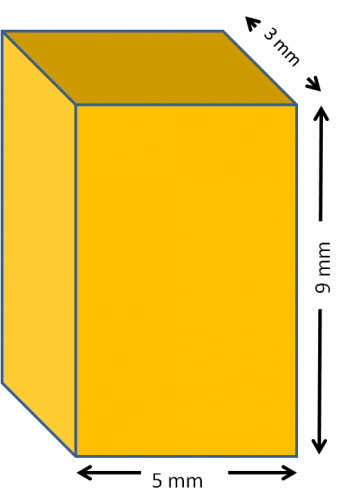The image above represents a cuboid.
To compute the area of a cuboid requires three essential parameters which are the length, width and height of the cuboid.

The formula for computing the area of a cuboid is:

A = 2(lw + lh + wh)

Where:
A = Area of the Cuboid
l = Length of the Cuboid
w = Width of the Cuboid
h = Height of the Cuboid

Let’s solve an example
Find the area of a cuboid with a length of 5 cm, width of 3 cm and a height of 9 cm.

This implies that:
l = length of the cuboid = 5
w = width of the cuboid = 3
h= = height of the cuboid = 9

A = 2(lw + lh + wh)
A = 2(5 x 3 + 5 x 9 + 3 x 9)
A = 2(87)
A = 174

Therefore, the area of the cuboid is 174 cm2.

Calculating the Length of a cuboid when Area, Width and Height are Given

The formula is l = A – 2(w)(h) / 2(w) + 2(h)

Where;
A = Area of the Cuboid
l = Length of the Cuboid
w = Width of the Cuboid
h = Height of the Cuboid

Let’s solve an example:
Find the length of a cuboid with an area of 140 cm2 , a width of 4 cm and a height of 12 cm

This implies that;
A = Area of the cuboid = 140 cm2
w = width of the cuboid = 4 cm
h  = height of the cuboid = 12 cm

l =  A – 2(w)(h) / 2(w) + 2(h)
l = 140 – 2(4)(12) / 2(4) + 2(12)
l = 140 – 96 / 8 + 24
l = 44 / 32
l = 1.375 cm

Therefore, the length of the cuboid is 1.375 cm.

Calculating the Width of a cuboid when Area, Length and Height are Given

The formula is w = A – 2(l)(h) / 2(l) + 2(h)

Where;
A = Area of the Cuboid
l = Length of the Cuboid
w = Width of the Cuboid
h = Height of the Cuboid

Let’s solve an example:
Find the width of a cuboid with an area of 200 cm2 , a length of 5 cm and a height of 12 cm

This implies that;
A = Area of the cuboid = 200 cm2
l = length of the cuboid = 5 cm
h  = height of the cuboid = 12 cm

w =  A – 2(l)(h) / 2(l) + 2(h)
w = 200 – 2(5)(12) / 2(5) + 2(12)
w = 200 – 120 / 10 + 24
w = 80 / 34
w = 2.353 cm

Therefore, the width of the cuboid is 2.353 cm.

Calculating the Height of a cuboid when Area, Length and Width are Given

The formula is h = A – 2(l)(w) / 2(l) + 2(w)

Where;
A = Area of the Cuboid
l = Length of the Cuboid
w = Width of the Cuboid
h = Height of the Cuboid

Let’s solve an example:
Find the height of a cuboid with an area of 300 cm2 , a length of 6 cm and a width of 2 cm

This implies that;
A = Area of the cuboid = 300 cm2
l = length of the cuboid = 6 cm
w  = width of the cuboid = 2 cm

h =  A – 2(l)(w) / 2(l) + 2(w)
h = 300 – 2(6)(2) / 2(6) + 2(2)
h = 300 – 24 / 12 + 4
h = 276 / 16
h = 17.25 cm

Therefore, the height of the cuboid is 17.25 cm.

Nickzom Calculator – The Calculator Encyclopedia is capable of calculating the area of a cuboid.

To get the answer and workings of the area of a cuboid using the Nickzom Calculator – The Calculator Encyclopedia. First, you need to obtain the app.

You can get this app via any of these means:

To get access to the professional version via web, you need to register and subscribe for NGN 1,500 per annum to have utter access to all functionalities.
You can also try the demo version via https://www.nickzom.org/calculator

Once, you have obtained the calculator encyclopedia app, proceed to the Calculator Map, then click on Mensuration under the Mathematics section.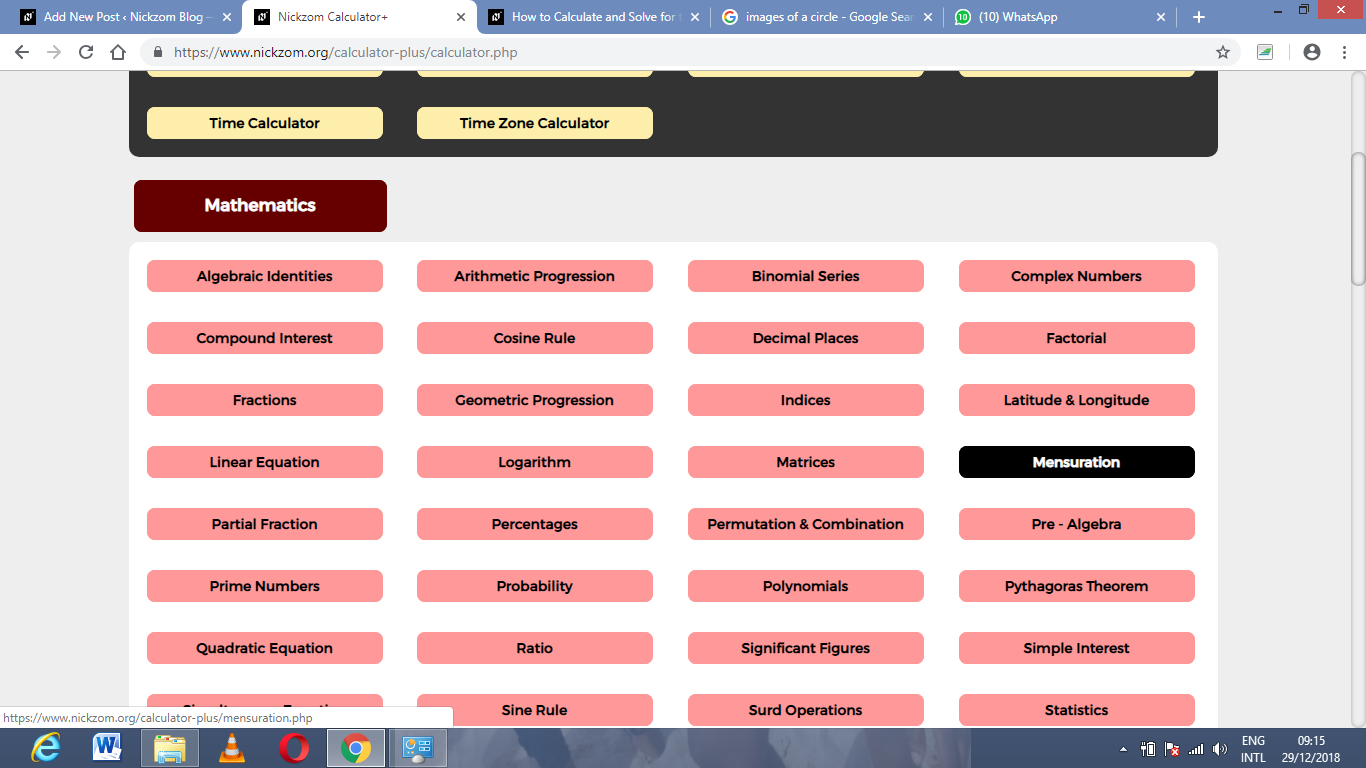Now, click on Area of a Cuboid under Mensuration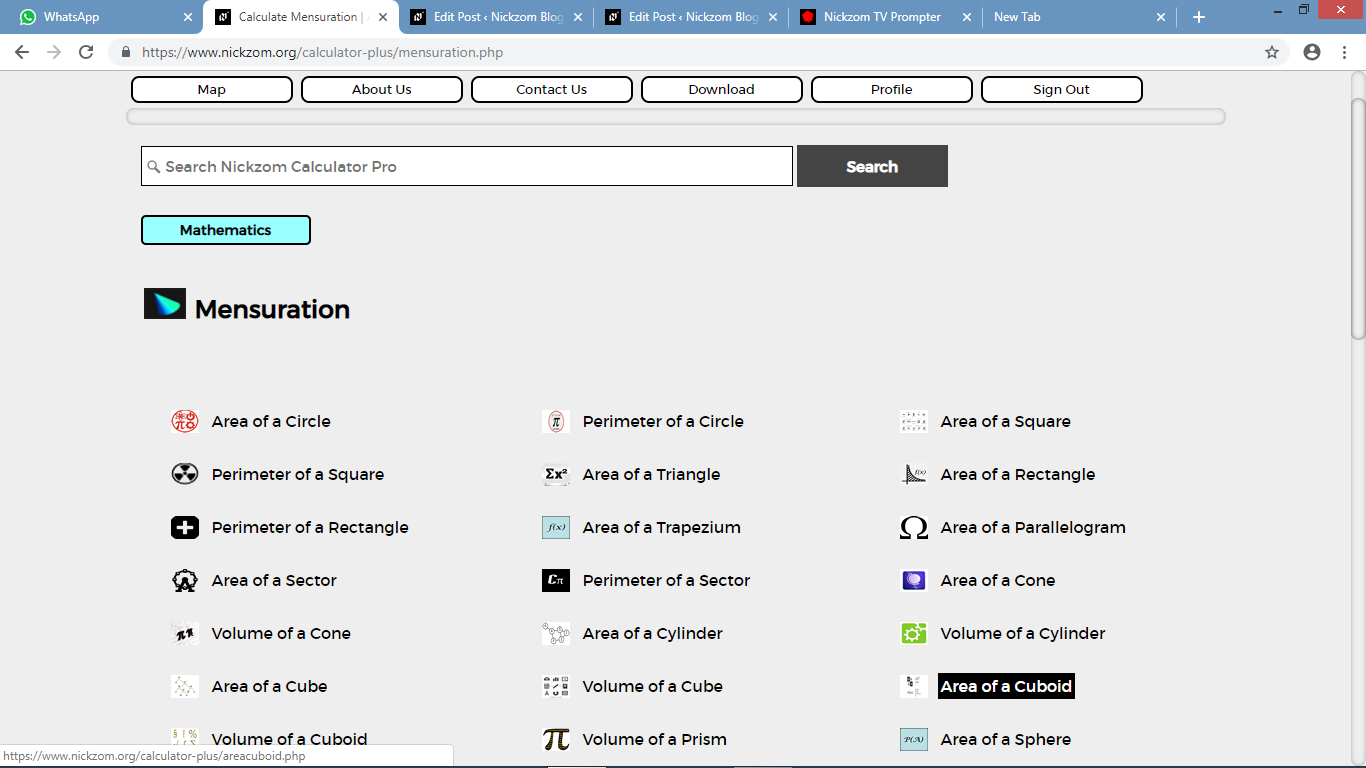The screenshot below displays the page or activity to enter your values, to get the answer for the area of a cuboid according to the respective parameters which are the width, length and height of the cuboid.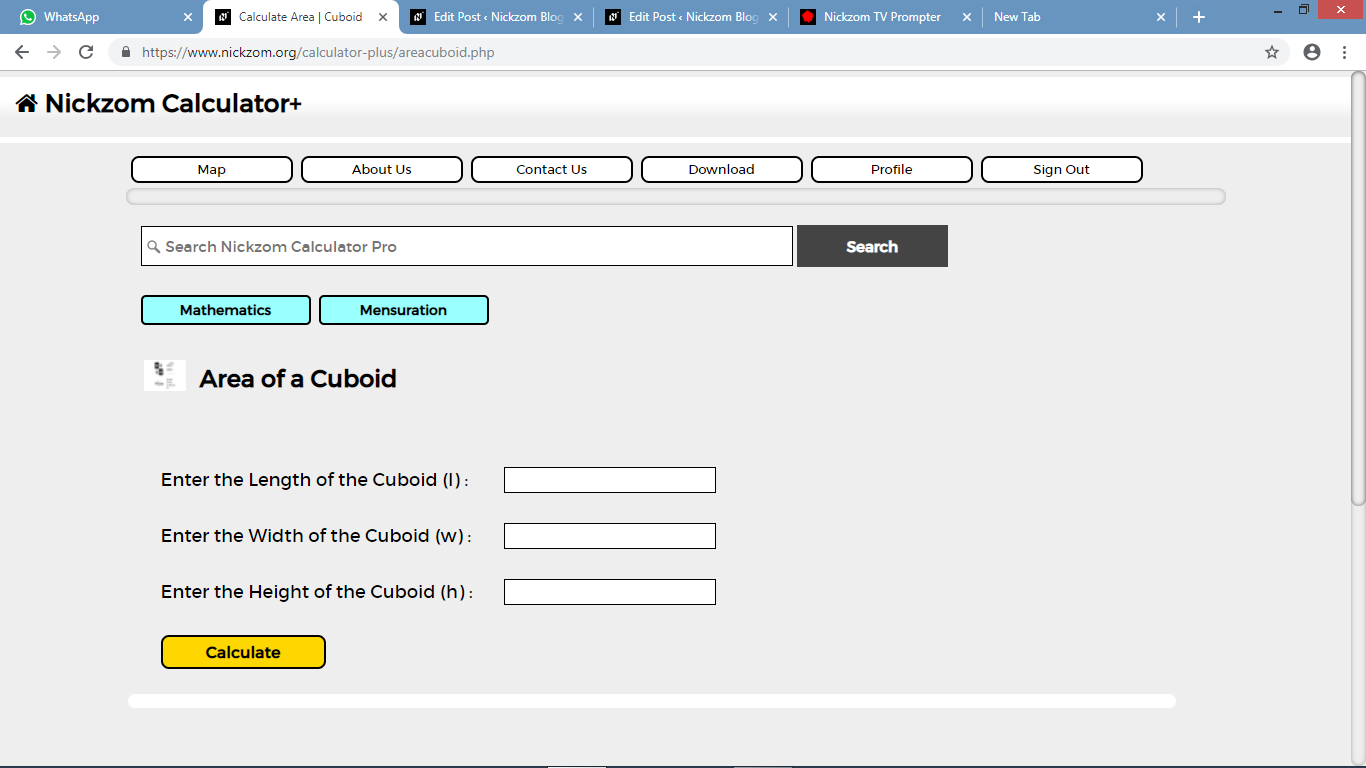Now, enter the values appropriately and accordingly for the parameters as required by the example above where the width of the cuboid is 3 cm, length of the cuboid is 5 cm and the height of the cuboid is 9 cm.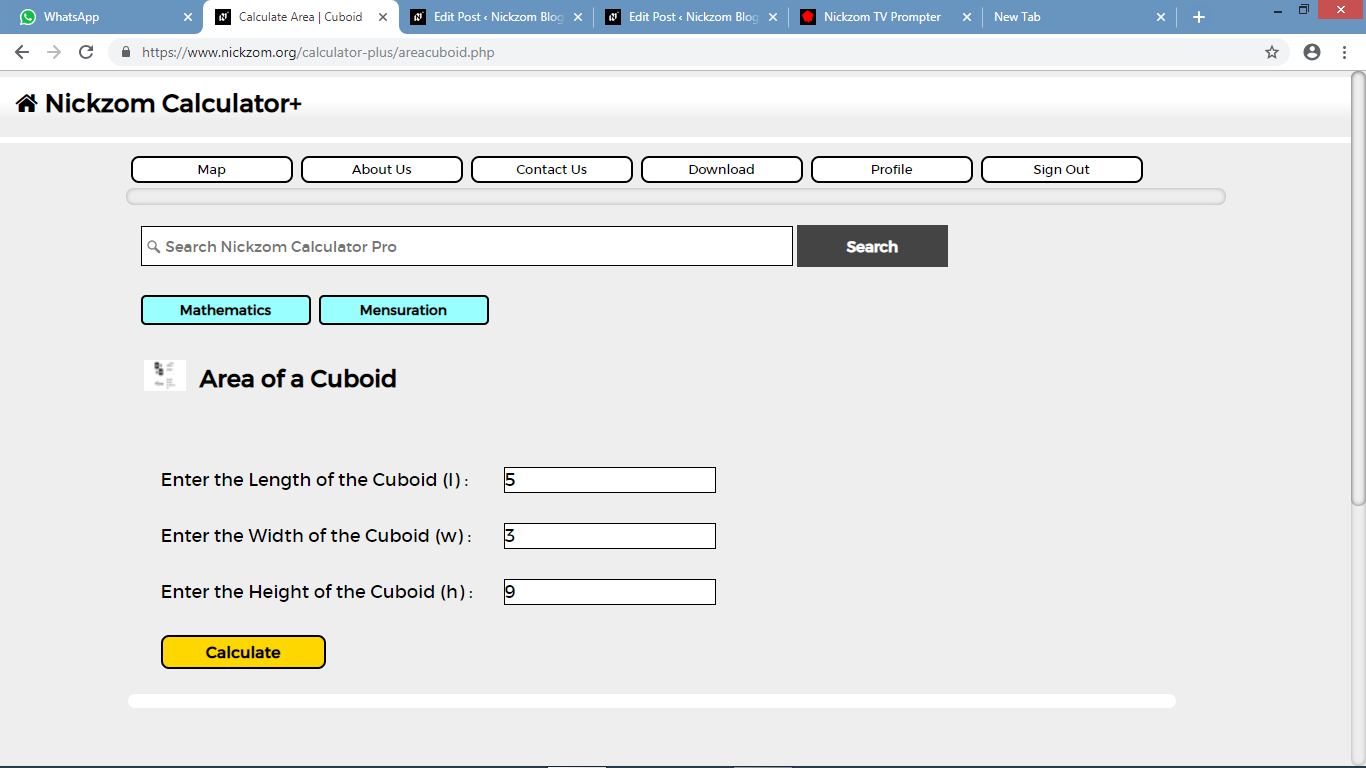Finally, click on the Calculate button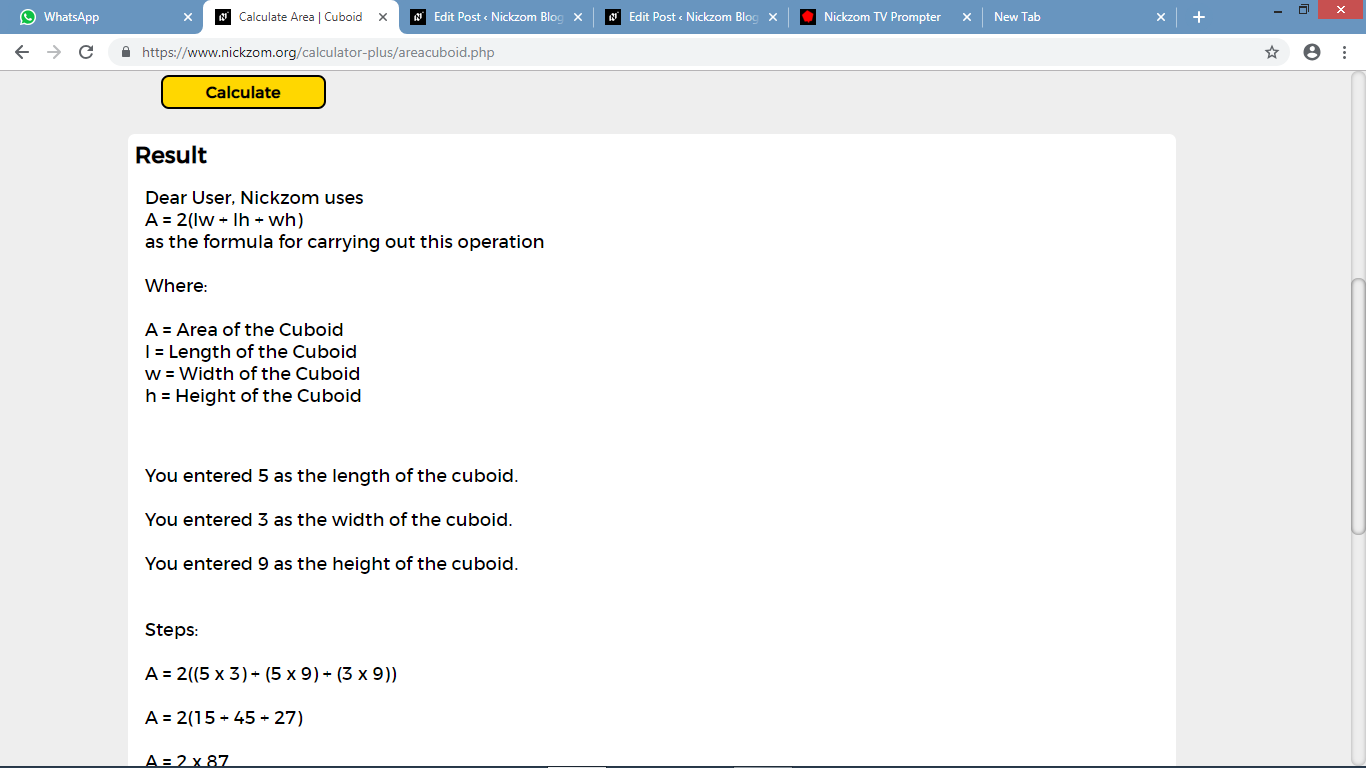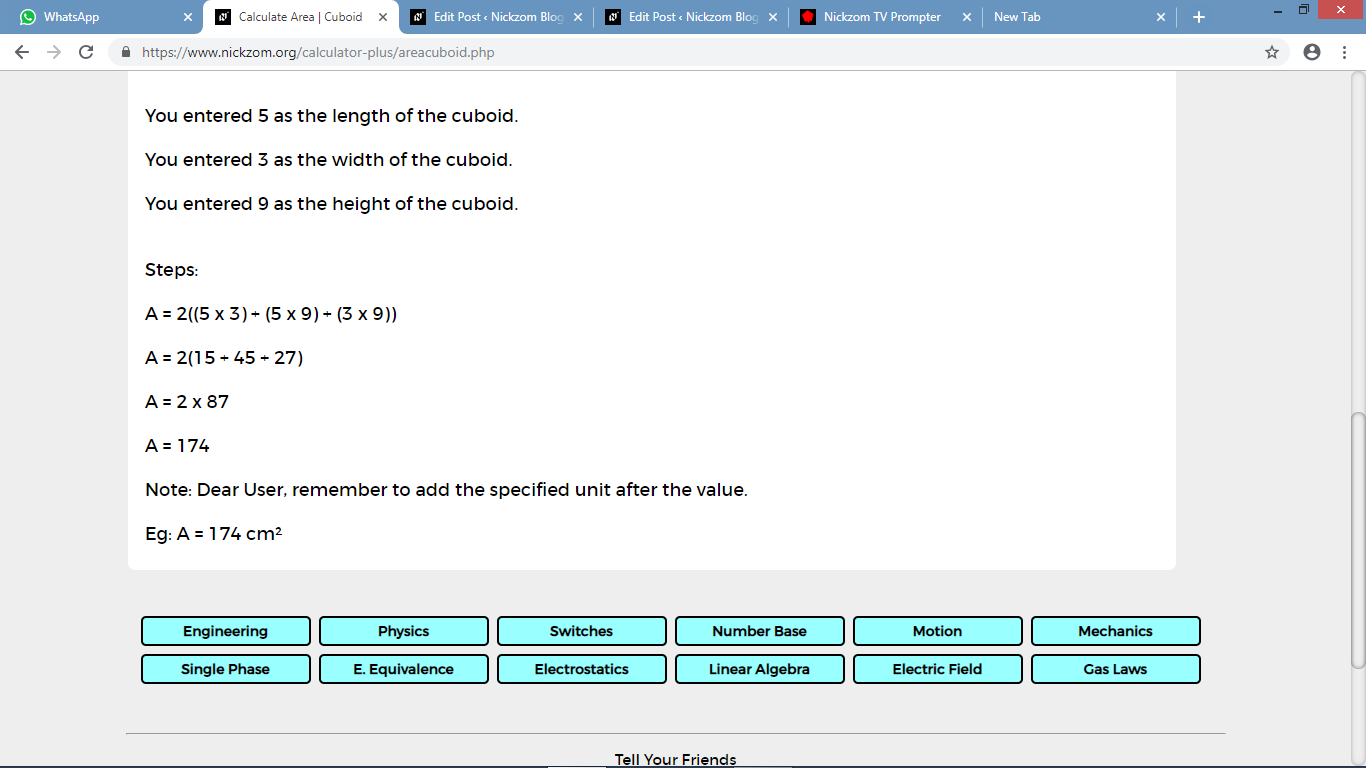As you can see from the screenshot above, Nickzom Calculator – The Calculator Encyclopedia solves for the area of a cuboid and presents the formula, workings and steps too.